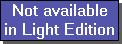The SDL Component Suite is an industry leading collection of components supporting scientific and engineering computing. Please visit the SDL Web site for more information....## PredictUnit: SDL_statis Class: TPLSModel Declaration:  procedure Predict (XData: TDoubleArray; var YHat: TDoubleArray); procedure Predict (XData: TMatrix; var YHat: TMatrix);

The method Predict allows you to predict unknown Y values once the model has been established by CalculateModel. Predict comes in two overloaded variants.

Version  estimates the response values YHat for a single object. Both the values of the independent variables XData and the resulting response data are passed as open arrays of double precision floating point numbers.

Version  calculates the response for several objects. The independent variables are passed in the array XData, the computed response data are returned in the matrix YHat.

In both versions the XData matrix has to have the same number of columns as the training data contained in XMat. The output structures YHat are automatically resized to fit the required number of returned estimates.

 Hint 1: Please note that the Predict method uses the regression coefficients of the model to estimate the responses. Thus the model has to be either calculated prior to the use of Predict, or the model has to be loaded from disk (using the method LoadModelCoefficients).

 Hint 2: When predicting a binary value (i.e. the property IsDiscriminantModel is TRUE) the returned predicted Y values have to be compared to the corresponding classifier threshold in order to obtain the binary estimate.

Last Update: 2020-Aug-18